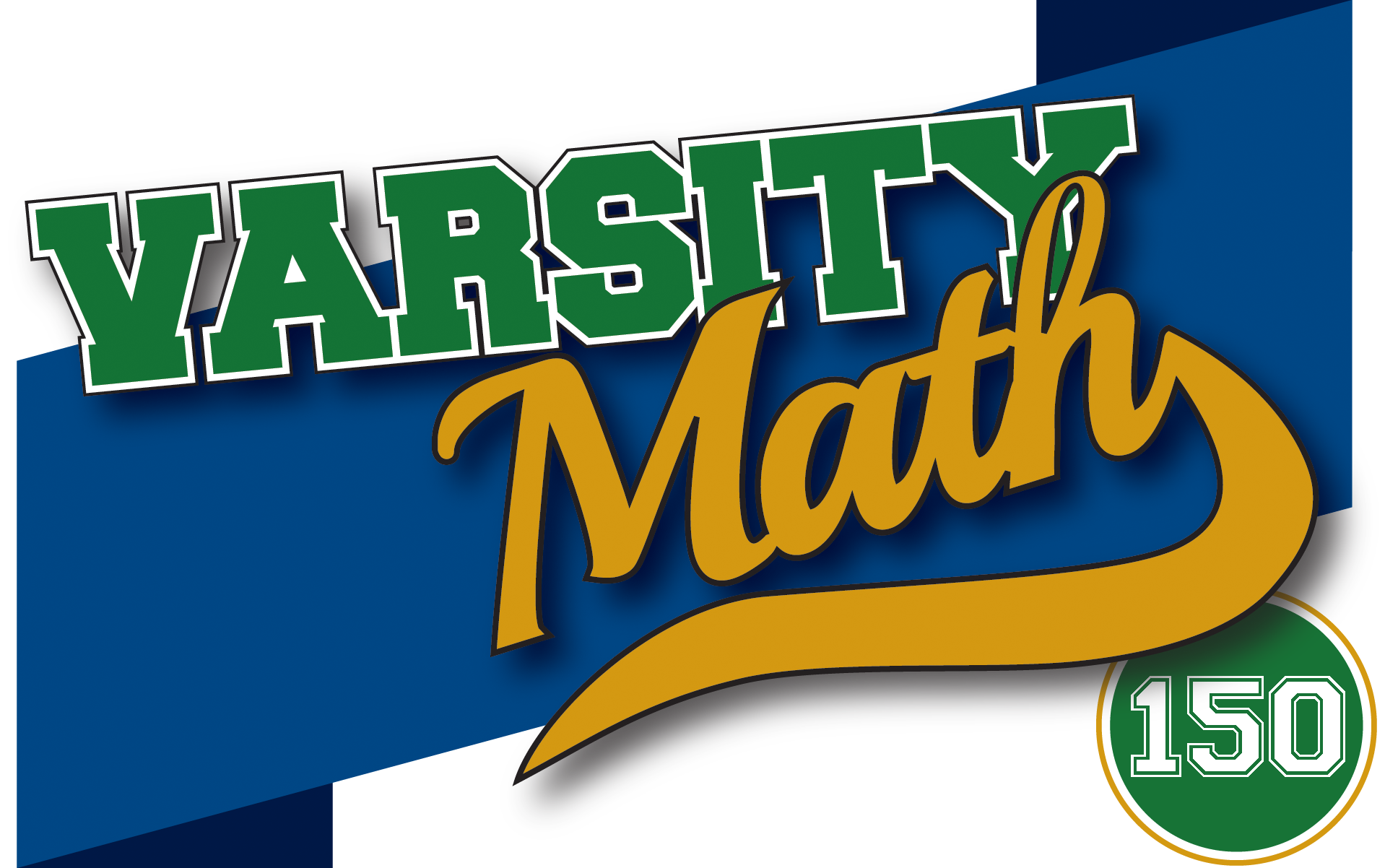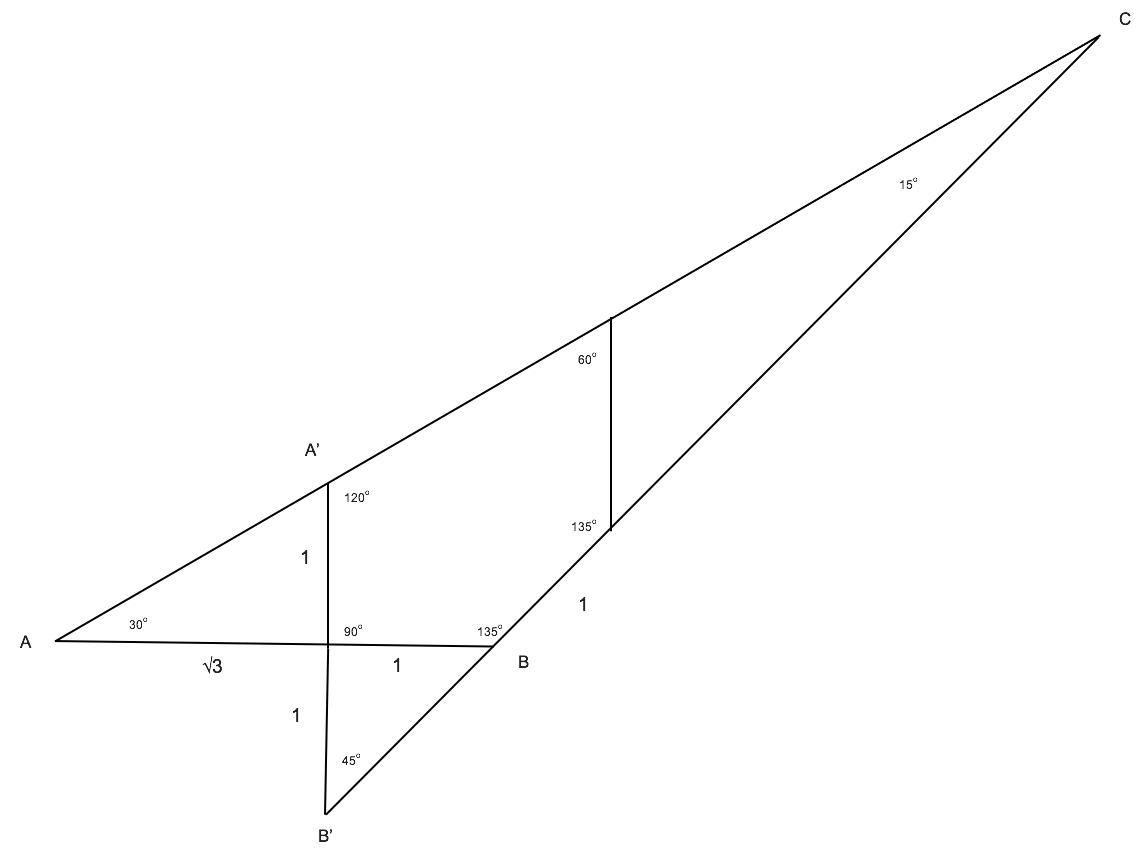## ________________

Welcome to Varsity Math, the weekly math puzzle column by the National Museum of Mathematics and featured each weekend in the Wall Street Journal.

## ________________### Make 29

Produce a mathematical expression equaling 29 in which you use the numeral 2 twice, 6 once, and +, -, ×, ÷, decimal points, and parentheses as many times as you wish.

### Circle of Friends

The math team members position themselves in a circle. Each is given a number and shows it to his or her two neighbors. Each then takes the average of the two numbers he or she was shown and announces the result. The announced numbers turn out to be 1, 2, 3, … and so on all around the circle in order. Cindy exclaims, “The number I was given initially is ten times the number I announced!”

How many members are in the circle if there are fewer than 30 in the group?

## Solutions to week 149

The solution to Tile the Square is shown in the figure below. In Largest Circle, the radius of the largest circle that can be drawn inside the polygon is r = (√3 – √2 + 1)/2 = .6589186…Draw the triangles ABC and A’B’C as shown. The largest circle within the polygon will be the smaller of the two inscribed circles of ABC and A’B’C. In ABC let c = AB = 1 + √3.

Then b = AC = sin135°/sin15°, a = sin30°/sin15° and the radius of the inscribed circle is r = .5 × (a + b – c)tan7.5° = (√3 – √2 + 1)/2 = .6589186…

In A’B’C let c’ = A’B’ = 2. Then b’ = A’C = c’sin45°/sin15°, a’ = c’sin120°/sin15° and the radius of the inscribed circle is r’ = .5 × (a’ + b’ – c’)tan7.5° = .6685466…

The smaller of the two radii is r = (√3 – √2 + 1)/2 = .6589186…## Recent Weeks

Links to all of the puzzles and solutions are on the Complete Varsity Math page.

Come back next week for answers and more puzzles.